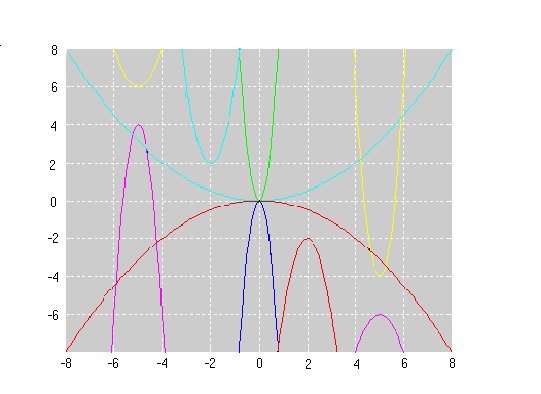#### You may also like### Cubic Spin

Prove that the graph of f(x) = x^3 - 6x^2 +9x +1 has rotational symmetry. Do graphs of all cubics have rotational symmetry?### Sine Problem

In this 'mesh' of sine graphs, one of the graphs is the graph of the sine function. Find the equations of the other graphs to reproduce the pattern.### Parabolic Patterns

The illustration shows the graphs of fifteen functions. Two of them have equations y=x^2 and y=-(x-4)^2. Find the equations of all the other graphs.

# Parabolas Again

##### Age 14 to 18Challenge Level

This problem was solved by Ali Abu Hijleh, age 13, Alex Liao, age 14 and Prateek Mehrotra, age 14 from Riccarton High School, Christchurch, New Zealand and Andrei Lazanu, age: 12 from School No. 205, Bucharest, Romania. Well done all of you. This is Andrei's solution:

I observed that the equations of the parabolas given in the problem are of the form: $y = a(x + b)^2 + c$

where positive corresponds to parabolas with the opening towards the top, and negative to parabolas with the opening towards the bottom; are the coordinates of the peak. I identified the parabolas in your pattern and I verified them with the help of a small program in Matlab:

x=-8:0.1:8

y1=-2*(x+6).*(x+6)-2

y2=-2*(x+4).*(x+4)-1

y3=-2*(x+2).*(x+2);

y4=-2*x.*x+1;

y5=-2*(x-2).*(x-2)+2;

y6=-2*(x-4).*(x-4)+3;

y7=-2*(x-6).*(x-6)+4;

y8=2*(x+6).*(x+6)+2;

y9=2*(x+4).*(x+4)+1;

y10=2*(x+2).*(x+2);

y11=2*x.*x-1;

y12=2*(x-2).*(x-2)-2;

y13=2*(x-4).*(x-4)-3;

y14=2*(x-6).*(x-6)-4;

plot(x,y1,x,y2,x,y3,x,y4,x,y5,x,y6,x,y7,x,y8,x,y9,x,y10,x,y11,x,y12,x,y13,x,y14)

hold

axis([-8 8 -4 4]);

grid

Consequently, the equations of the 14 parabolas in your pattern are:

$y = -2(x + 6)^2 - 2$

$y = -2(x + 4)^2 - 1$

$y = -2(x + 2)^2$

$y = -2x^2 + 1$

$y = -2(x - 2)^2 + 2$

$y = -2(x - 4)^2 + 3$

$y = -2(x - 6)^2 + 4$

$y = 2(x + 6)^2 + 2$

$y = 2(x + 4)^2 + 1$

$y = 2(x + 2)^2$

$y = 2x^2 - 1$

$y = 2(x - 2)^2 - 2$

$y = 2(x - 4)^2 - 3$

$y = 2(x - 6)^2 - 4$

I created my own pattern with a Matlab program and here are the graphs:Andrei supplied the Matlab program but in order to challenge you to find his equations it is not reproduced here